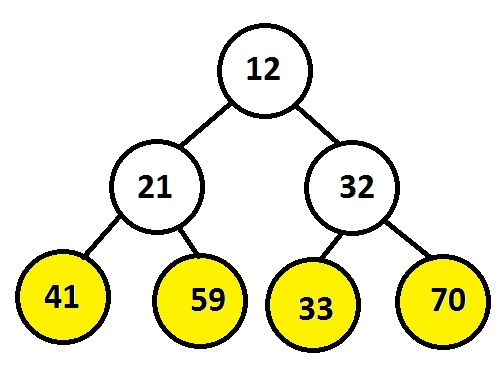# Print leaf nodes in binary tree from left to right using one stack in C++

Program should print the leaf nodes of a binary tree from left to right but the challenge involves is using of only one stack

Through push() function insert nodes of a binary tree and through pop() operation display the leaf nodes.

Leaf nodes are the end nodes whose left and right pointer is NULL which means that particular node is not a parent node.

## Example

Input : 12 21 32 41 59 33 70
Output : 41 59 33 70Stack is a data structure which is a LIFO structure in which top pointer will point to the last elements inserted so the leaf nodes will be inserted at the last into the stack and they will be pop out or removed from the stack before any other node as per stack structure.

The below code shows the C++ implementation using STL of the algorithm given.

## Algorithm

START
Step 1 -> create node variable of type structure
Declare int data
Declare pointer of type node using *left, *right
Step 2 -> create function for inserting node with parameter as val
Declare node variable of node using malloc
Set node->data = val
Set node->left = node->right = NULL
return node
step 3 -> Declare Function void leaf(Node *ptr)
create vector stack<Node*>stck
Loop While 1
IF ptr
Stck.push(ptr)
Ptr = ptr->left
Else
IF (stck.empty())
Break
Else
IF (stck.top()->right == NULL)
Set ptr = stck.top()
Set stck.pop()
IF ptr->left = NULL
Print ptr->data
End
Loop While ptr == stck.top()->right
Set ptr = stck.top()
Call stck.pop()
IF stck.empty()
Break
End
IF !stck.empty()
Set ptr = tck.top()->right
Else
Set ptr = NULL
EndIF
End
End
End
Step 4-> In main()
Call New passing value user want to insert as Node* root = New(12)
Call leaf(root)
STOP

## Example

#include <bits/stdc++.h>
using namespace std;
// Structure of a node
struct Node {
Node* left;
Node* right;
int data;
};
//Function to create a new node
Node* New(int val) {
Node* node = new Node();
node->left = node->right = NULL;
node->data = val;
return node;
}
// leaf node using stack
void leaf(Node* ptr) {
// stack that will store nodes
stack<Node*> stck;
while (1) {
if (ptr) {
stck.push(ptr);
ptr = ptr->left;
} else {
if (stck.empty())
break;
else {
if (stck.top()->right == NULL) {
ptr = stck.top();
stck.pop();
// Print the leaf node
if (ptr->left == NULL)
printf("%d ", ptr->data);
}
while (ptr == stck.top()->right) {
ptr = stck.top();
stck.pop();
if (stck.empty())
break;
}
if (!stck.empty())
ptr = stck.top()->right;
else
ptr = NULL;
}
}
}
}
int main() {
printf("leaf nodes at end level are : ");
Node* root = New(12);
root->left = New(21);
root->right = New(32);
root->left->left = New(41);
root->left->right = New(59);
root->right->left = New(33);
root->right->right = New(70);
leaf(root);
return 0;
}

## Output

If we run above program then it will generate following output.

leaf nodes at end level are : 41 59 33 70